Home CHEMISTRY TOPIC 7: FORMULA BONDING AND NOMENCLATURE | CHEMISTRY FORM 2

# TOPIC 7: FORMULA BONDING AND NOMENCLATURE | CHEMISTRY FORM 2

1058
1### Valence and Chemical Formulae

The Concept of Valence
Explain the concept of valence
Valency is the capacity of an atom to combine with one or more atoms to form a molecule or compound. Valency also refers the number of electrons that an atom can gain, lose or share in forming a chemical bond with another atom.
The valency (or combining power) depends on the number of
electrons in the outermost orbit (or valency shell) involved in the
formation of a chemical bond. The number of electrons in the valency
shell is never greater than 7. The outermost electronic configuration is
responsible for the variability of the valency.
Some elements exhibit more than one valency, i.e., they have variable
valencies. Examples of elements with variable valencies are iron (2 and
3), tin (2 and 4) and copper (1 and 2). The other elements with variable
valencies are as shown in table 7.1.
Valency and Oxidation States
There is a strong correlation between valency and oxidation state. The
oxidation state of an element equals its valency or charge carried by
its ion when an element ionizes in solution. An example of this relation
is iron (II) whose oxidation state (or oxidation number) is 2 and its
valency is 2. The same case applies to iron (III).
Other elements with variable valencies such as copper (I) and copper (II) have oxidation state equal to 1 and 2 respectively. The list continues. You will learn more about oxidation states later.
The valencies of the common transition elements should be memorized.
Valencies of the normal elements may be deduced from the group number
they occupy in the Periodic Table. The valencies of elements of group I
to IV are equal to the group numbers they occupy in the periodic table.
The valency of an element in group V to VIII is equal to eight minus the
group number. For example, the valency of chlorine which is in group
VII is 1, i.e. (8 -7) =1. The valency of oxygen in group VI is 2, i.e.
(8-6) =2. Elements in group 0 (or VIII) have zero valency i.e. (8 – 8) =
0.
Table 7.1. Valencies of common metals and non-metals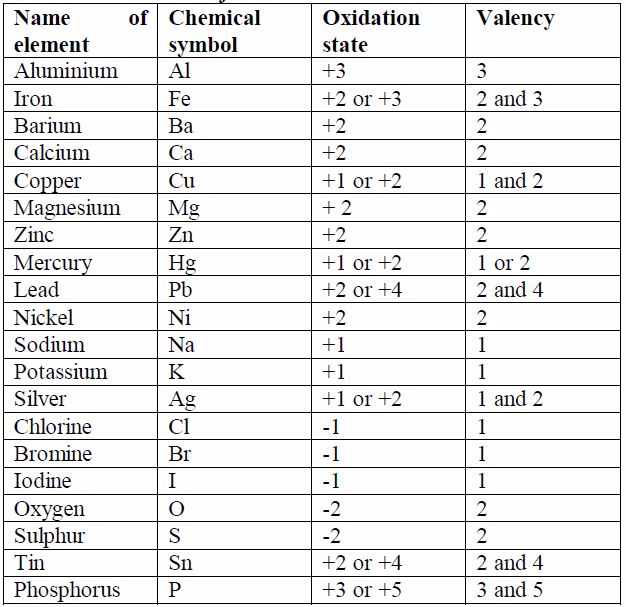Simple Formulae of Binary Compounds
Write simple formulae of binary compounds
Chemical Formulae
The
Chemical formula is a method of representing the molecule of a compound
by using chemical symbols. It is a way of expressing information about
the atoms that constitute a particular chemical compound. The chemical
formula identifies each constituent element by its chemical symbol and
indicates the number of atoms of each element found in each single
molecule of that compound.
The
symbol for hydrogen atom is H. When two hydrogen atoms join together
they form a molecule,H2. The number 2 to the right and below the symbol
shows the number of atoms a molecule contains. P4 and S8 represents 4
atoms of phosphorus and 8 atoms of sulphur contained in one molecule of
phosphorus and one molecule of sulphur respectively.
While
the formula for chlorine molecule isCl2, it cannot be expressed as 2Cl.
This is because 2Cl means two atoms of chlorine and not a molecule of
chlorine.H 2 O stands for a molecule of water which consists of two
hydrogen atoms and one oxygen atom.
H2SO4stands
for a molecule of sulphuric acid containing 2atoms of hydrogen, 1 atom
of sulphur and 4 atoms of oxygen.CaCO3 stands for a molecule of calcium
carbonate containing 1atom of calcium, 1 atom of carbon and 3 atoms of
oxygen.Where it deems necessary to show the number of molecules a
compound contains, this is achieved by writing the appropriate number
before the formula of the compound. A few examples are shown below:

2H 2O means two molecules of water

3H2 SO4 means three molecules of sulphuric acid

5CaCO3 means five molecules of calcium carbonate

It
is important to note that the figure appearing before the formula
multiplies the whole of it. For example, 3H2SO4 stands for 3 molecules
of sulphuric acid containing six atoms of hydrogen, three atoms of
sulphur and twelve atoms of oxygen.It is a big mistake to think that the
number 3 before the molecule multiplies only the symbol which
immediately follows it (that is,H2). This is quite wrong. The 3
multiplies the whole of the formula
Formulae of Binary Compounds
A
binary compound is a compound made of only two types of the reacting
species, for example, sodium chloride (NaCl),which is made of only
sodium and chlorine, is a binary compound. Look at table 7.1. The size
of the charge on an ion isa measure of its valency or combining power.
You will notice that, ignoring the signs for the charge on ions, the
value of the charge on ion is equal to the valency of the atom. You will
need to memorise the valencies of these metals as much as possible so
as to be able to write the formulae of their compounds correctly.
The following are the rules for writing down the chemicalformulae of chemical substances:

Metals (or their positively charged ions) must start in theformula, followed by non-metals (or their negatively chargedions).

Where the formula is to include a radical, the radical must betreated as a single atom and must be bracketed if need be.

Ammonium ion is to be treated as if it were a metal.

Positive charges must be equal to negative charges for aneutral molecule or compound.

Single elements; say Na, K, Si, Ag, etc. should not bebracketed.

Valencies of metals (or positive ions) should be exchangedand written as subscripts.

The valency of 1 is simply assumed and not written in theformula.

This
is best shown by using some examples. The following procedure must be
followed when writing the formulae of binary compounds:

Write down correct symbols for atoms of elements or ions that make up the compound.

Write down the valencies of the atoms of the elements.

Interchange
the valencies and write them as subscripts in the final formula of the
compound. Remember that the valency of 1 is not expressed in the
formula. At this final step, the radicals must be bracketed if
necessary.

Study the following examples and make sure you understand how this works:
Write the formula for aluminium oxide: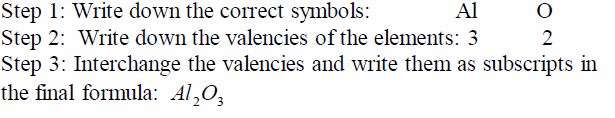Write down the formula for water: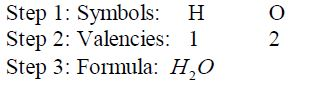Formula for sodium nitrate: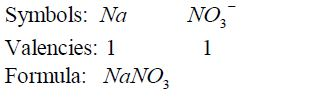Formula for calcium nitrate: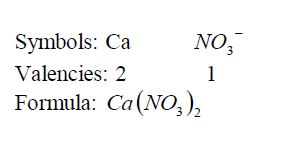Formula for ammonium nitrate: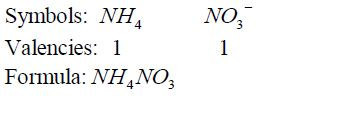Formula for calcium oxide: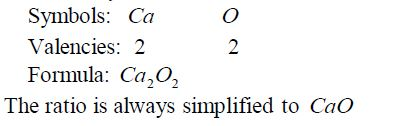Formula for aluminium sulphate: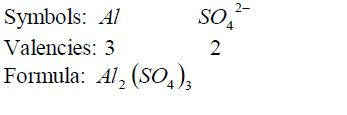Ammonia: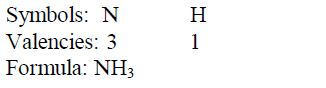Carbon dioxide: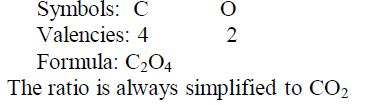Nomenclature of Inorganic Compounds
The
term “nomenclature” refers to “system of naming”. The system of naming
in use is that recommended by the IUPAC (International Union of Pure and
Applied Chemistry). The modern system of naming reveals the type of
elements present in a given compound. The old or trivial names have been
dropped out.
Some
common and important compounds have historical names that do not seem
to fit in the system, for example water H O 2 ,ammonia 〈NH3〉, methane ,
CH4and mineral acids such as sulphuric (VI) acid〈H2SO4〉, nitric (V)
acid〈HNO3〉and hydrochloric acid〈HCl〉. Also organic acids such as
ethanoic acid (CH3COOH) are also included in this group. These names are
trivial but they have been adopted in modern nomenclature.If these
exceptions are omitted, there are basic generalizations that are useful:

If
there is a metal in the compound, it must be named first. In this case
ammonium ion, NH4¹, is regarded as if it were a metal in the compounds
it occurs such as NH4 NO3 , NH Cl 4 , etc.

For elements with
variable valencies such as iron and lead,Roman numerals are included in
the name to indicate the valency of the metal or the ion which is
present. For example,iron (III) chloride contains Fe3+ while iron (II)
chloride contains Fe² . The same case applies to lead (II) and lead
(IV)compounds and so on.

Compounds containing two elements only
(binary compounds) have names ending in …..ide; for example sodium
chloride (NaCl), calcium bromide (CaBr2), magnesium nitrite( Mg3 N2) ,
etc. The important exception to this is hydroxides,which contains the
hydroxide (OH) ion.

Compounds containing a poly atomic ion
(usually containing oxygen) have names that end with …ate; for example
calcium carbonate (CaCO3) , potassium nitrate(KNO3) , magnesium
sulphate(MgSO4) , sodium ethanoate (CH3 COONa) , etc.

The names
of some compounds use prefixes to tell you the number of that particular
atom in the molecule. This is useful if two elements form more than one
compound. For example:carbon monoxide (CO), carbon dioxide (CO2),
nitrogen dioxide NO2 dinitrogen tetra oxide N2 O4 , sulphur dioxide SO2
sulphur trioxide SO3 , etc.

The
following prefixes indicate the number of atoms in caseslike this: mono
– one; di – two; tri – three; tetra – four; pent –five; hex – six; hept
– seven; oct – eight; non – nine; and dec –ten.
The Concept of Empirical and Molecular Formulae
Explain the concept of empirical and molecular formulae
The
empirical formula is the simplest formula of any compound.It expresses
the simplest ratio of all the atoms or ions that makeup a certain
compound. For example, the empirical formula ofthe compound with the
formula, C2H4is CH2. This means thatthe simplest ratio of (C:H) is 1:2.
This ratio also indicates theratio in which carbon and hydrogen atoms
combine to form thecompound C2H4.
The
molecular formula is the formula which shows the actual number of all
atoms present in a given compound. For example,the molecular formula of
the above compound is C2H4. This means that two atoms of carbon and four
atoms of hydrogen form the compound. Likewise, the molecular formula of
water isH2O meaning that the compound is made up of two atoms of
hydrogen and one atom of oxygen.
Therefore, the empirical and molecular formulae can each bedefined thus:
The empirical formula
of a compound is the simplest formula which shows its composition by
mass, and which shows the ratio of the number of the different atoms
present in the molecule.
The molecular formula of a compound is the one which showsthe actual number of each kind of atom present in its molecule.
The
empirical formula differs from the molecular formula of the same
compound since only the molecular formula agrees with the molar mass of
the compound.
Information given by Empirical and Molecular Formulae
The formula for water is H 2 O . From this information, you can see that:

2 hydrogen atoms combine with 1 oxygen atom to form one molecule of water.

moles
of hydrogen atoms combine with 1 mole of oxygen atoms. Moles can be
changed to grams using relative atomic masses (RAMs). So we can write:

grams of hydrogen combines with 16 grams of oxygen. In the same way:

1g of hydrogen combines with 8g of oxygen.

4g of hydrogen combines with 32 of oxygen.

The
masses of each substance taking part in the reaction are always in the
same ratio.Therefore, from the molecular formula of a compound you can
tell:

how many moles of different atoms combine;

how many grams of the different elements combine;

the number of each kind of atoms of different elements that combine to make up a compound; and

the percentage of each atom in a compound based on RAMs of each atom And from the empirical formula you can tell:

the simplest ratio or proportion of the different atoms that combine to form a compound.

Example 1
the
empirical formula of ethane C2H4andpropene C3H6with molar masses 28.0g
and 42.0grespectively is CH2(i.e. the same) although the two compounds
possess different molecular formulae and masses.
In
general, the empirical formula multiplied by a whole number,n, gives
the molar mass of the compound. So long as the value of n is known, then
the molecular mass can be deduced.For example, suppose the molecular
mass of ethene is 28.0g, its molecular formula can be deduced thus: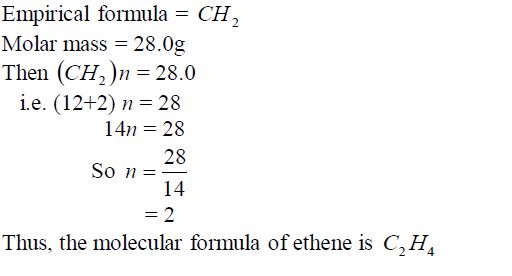Similarly,
suppose carbon dioxide has a molar mass of 44g and its empirical
formula is CO2. Its molecular formula can be determined thus: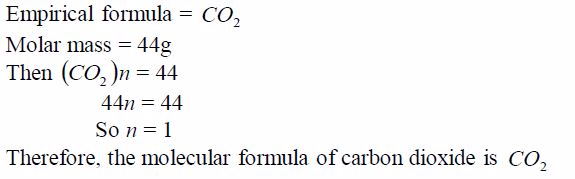The Empirical and Molecular Formulae
Calculate the empirical and molecular formulae
The Empirical Formula
Chemists
find the percentage by mass of each element in a compound by
experiment. Using this information, it is then possible to find the
simplest formula of that compound. To do this we shall also need to know
the relative atomic mass of each element present in the compound.
An experiment to find the empirical formula of a compound
To
work out the empirical formula you need to know the masses of elements
that combine. For example, magnesium combines with oxygen to form
magnesium oxide. The masses that combine can be found like this:
Procedure

Weigh a crucible and lid, empty. Then add a coil of magnesium ribbon and weigh again.

Heat the crucible. Raise the lid carefully at intervals to let oxygen in. The magnesium ribbon burns brightly.

When
burning is complete, let the crucible cool (still with its lid on).
Then, weigh again. The increase in mass is due to oxygen.

Results
Here are sample results and the calculation:

Mass of crucible + lid = 25.2 g

Mass of crucible + lid + magnesium = 27.6g

Mass of crucible + lid + magnesium oxide = 29.2g

Mass of magnesium = 27.6g – 25.2g = 2.4g

Mass of magnesium oxide = 29.2g – 25.2g = 4.0g

Mass of oxygen, therefore = 4.0g – 2.4g =1.6g

Therefore,
2.4g of magnesium combines with 1.6g of oxygen.The RAMs are Mg = 24, O =
16. Changing masses to moles:24/2.4moles of magnesium atoms combines
with1.6/16 moles of oxygen atoms
0.1
moles magnesium combines with 0.1moles of oxygen atoms.So the atoms
combine in a ratio of 0.1:0.1 or simply 1:1The empirical formula of
magnesium oxide = MgO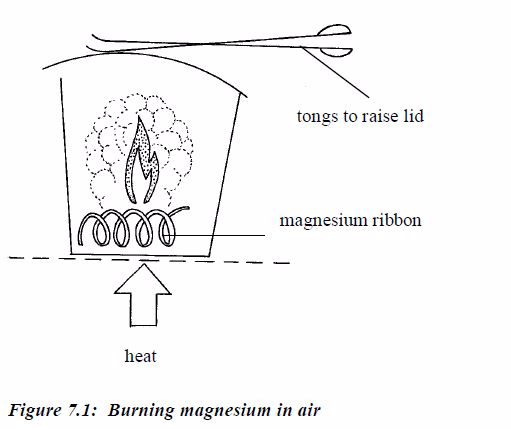Sometimes
the empirical formula can be determined from the provided percentage
composition or weight of atoms of the elements that constitute a
compound.
Example 2
An
experiment shows that 32g of sulphur combines with 32g ofoxygen to form
the compound sulphur dioxide. What is itsempirical formula?
Solution
Divide
each mass by the RAM of the respective element. This gives the ratio of
different numbers of atoms of each element that make up the compound.
Again, divide each of these ratios by the smallest to get the whole
number ratio. This gives the simplest ratio of the constituent elements
possible. Sometimes you may not get a whole number ratio. If this
happens, round off the ratio to the nearest whole number. Finally, write
down the formula using the obtained ratio of the elements. Study the
following table and make sure you understand the procedure: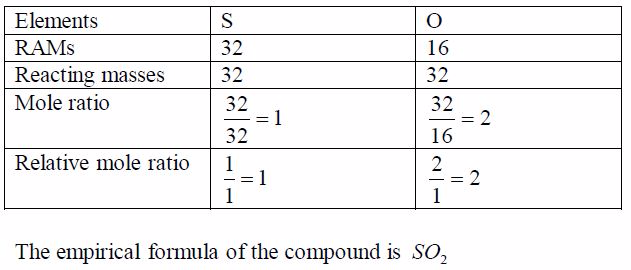Example 3
An
oxide of carbon contains 27.3% carbon. Find the empirical formula of
the oxide.Think big!What other element is present in the oxide of
carbon?How do you know that the percentage of this other element
is72.7%?
Solution
In
order to work out the simplest formula we divide each percentage by the
relative atomic mass of each element. This allows a comparison of the
different numbers of atoms of each element that are present. We get a
ratio of each element with respect to each other as worked out in the
table below. To get a whole number ratio, we again, divide each of these
ratios by the smallest.The result shows the simplest ratio of atoms, in
this case one carbon atom to two oxygen atoms. The simplest ratio,
therefore,is CO2 .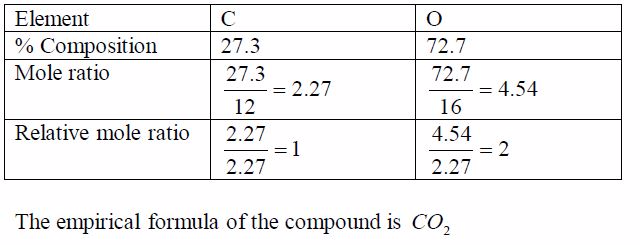Example 4
A
compound X is a hydrocarbon. It contains only carbon and hydrogen
atoms. 0.84g of X was completely burned in air. This produced 2.64g of
carbon dioxide CO2 and 1.08g of water H2 O. Find the empirical formula
of X.
Solution

In CO2 ,12/44of the mass is carbon

All the carbon came from X

So
2.64g of CO2 contains 2.64g〈12/44〉*2.64g= 0.72g of carbon
and,therefore, 0.12g of hydrogen (0.84g – 0.72g = 0.12g)Since we have
deduced the weights of the respective elements in the compound, we can
now work out the empirical formula as usual: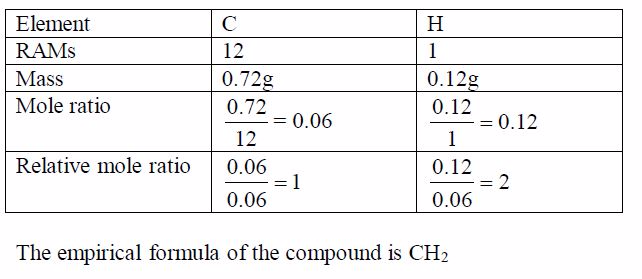The Molecular Formula
The
molecular formula is more useful than the empirical formula because it
the same. For others they are different.
To find the molecular formula of an unknown compound youneed to know:

the empirical formula; and

the molecular weight of the compound.

Once you have these two pieces of information you can now work out the molecular formula.
Example 5
A compound contains 85.7% carbon and 14.3% hydrogen. Itsmolecular weight is 28. What is its molecular formula?
Solution
Step 1: Find the empirical formula of the compound: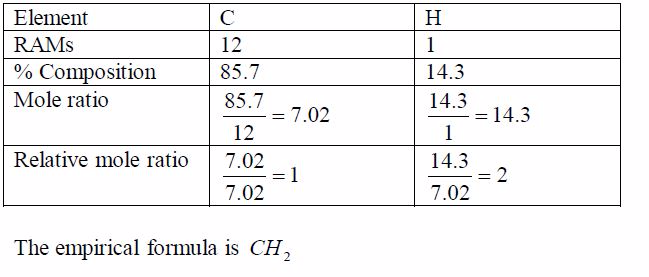Step 2: Find the molecular formula using the relation   2 CH n =Molecular formula: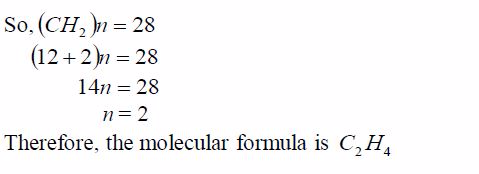Example 6
A compound contains 43.4% sodium, 11.3% carbon and 45.3%oxygen.
(a) Find the empirical formula of the compound.(b) If its molecular weight is 106, calculate its molecularformula.
Solution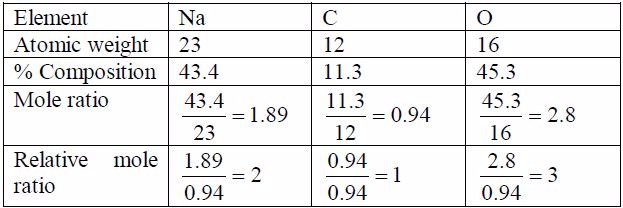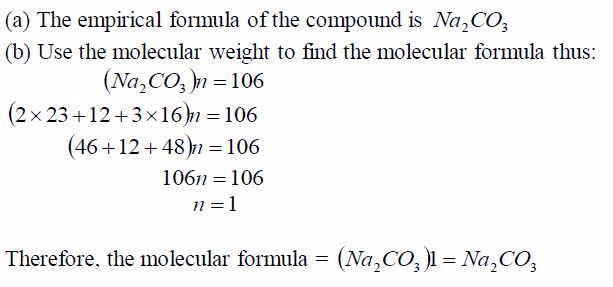In
some cases, the molecular weights are given while in others the vapour
density is given. To get the molecular weight,multiply the vapour
density by two, e.g.:Molecular weight = Vapour density  2
Example 7
A hydrocarbon has a vapour density of 15, and it contains 20%by mass of hydrogen. Calculate the formula of the hydrocarbon.
Solution
If H = 20%, then C = 80%
Use these values to find the relative number of moles of atoms present: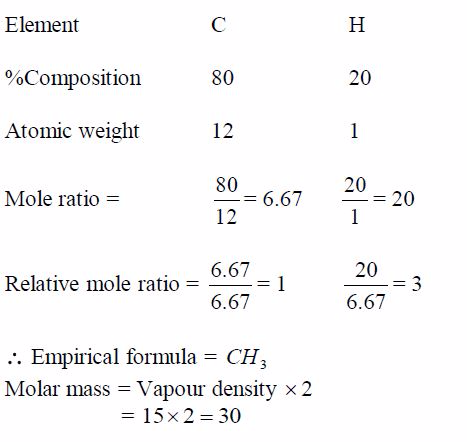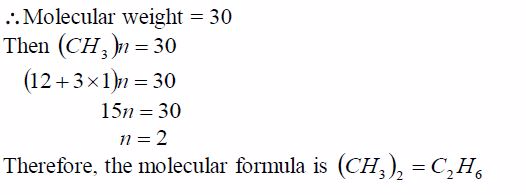#### 1 COMMENT

1. Most people have no clue that scalp therapy shampoos for fast hair growth (obviously with no sulfates, no parabens and no DEA) are even a thing. Persons are now able to enjoy longer hair and attain more options. Certainly worth investigating.

Whether you’re studying alopecia, damaged hair, avoiding hair disorders, hair growth, hair and scalp health in general, similar ideas apply.

Generally, you will want to steer clear of hair products and treatments that include chemicals such as parabens, DEA and sulfates.

What’s healthy for your hair is healthy for your skin all the same.

Clearly your content here is so useful for various reasons. It avoids the accustomed traps and pitfalls most fall into: getting defective alternatives. Greatly appreciated!